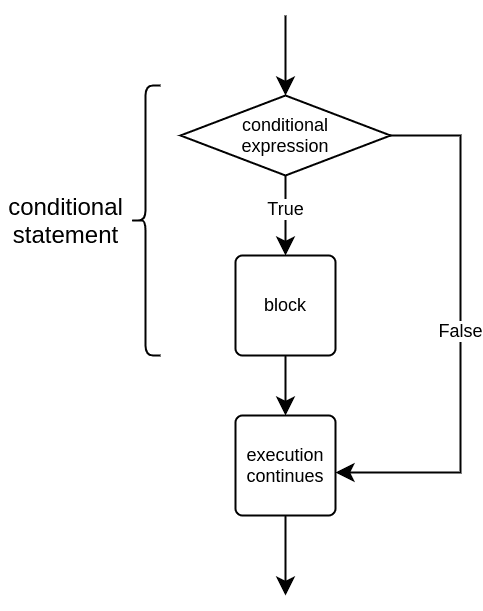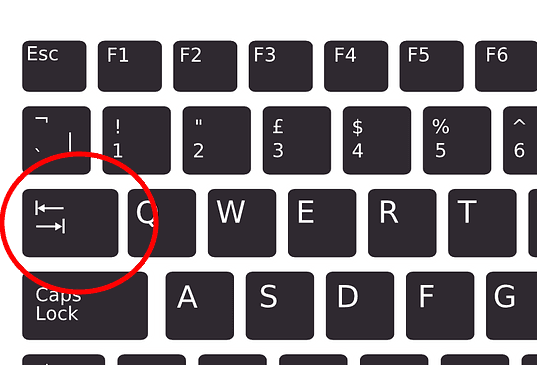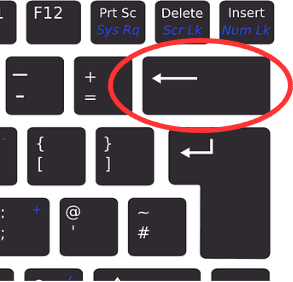Part 1

# Conditional statements

Thus far, every program we have written has been executed line by line in order. Instead of executing every line of code every single time a program is run, it is often useful to create sections of the program which are are only executed in certain situations.

For example, the following code checks whether the user is of age:

``````age = int(input("How old are you? "))

if age > 17:
print("You are of age!")
print("Here's a copy of GTA6 for you.")

When the user is over the age of 17, the execution of the program should look like this:

Sample output

How old are you? 18 You are of age! Here's a copy of GTA6 for you. Next customer, please!

If the user is 17 or under, only this is printed out:

Sample output

How old are you? 16 Next customer, please!

These examples show us that the value given as input affects which parts of the program are executed. The program contains a conditional statement with a block of code which is executed only if the condition in the statement is true.In a conditional statement the keyword `if` is followed by a condition, such as a comparison of two values. The code block following this header line is only executed if the condition is true.

Notice the colon character following the `if` header. In the following code there is no colon:

``````age = 10

# no colon at the end of the following line
if age > 17
print("You are of age.")``````

Upon execution this causes an error:

Sample output
```File "program.py", line 3
if age > 17
^
SyntaxError: invalid syntax
```

## Comparison operators

Very typically conditions consist of comparing two values. Here is a table with the most common comparison operators used in Python:

OperatorPurposeExample
`==`Equal to`a == b`
`!=`Not equal to`a != b`
`>`Greater than`a > b`
`>=`Greater than or equal to`a >= b`
`<`Less than`a < b`
`<=`Less than or equal to`a <= b`

Let's have a look at a program which prints out different things based on whether the number the user inputs is negative, positive, or equal to zero:

``````number = int(input("Please type in a number: "))

if number < 0:
print("The number is negative.")

if number > 0:
print("The number is positive.")

if number == 0:
print("The number is zero.")``````

Examples of how the program functions with three different inputs:

Sample output

Please type in a number: 15 The number is positive.

Sample output

Please type in a number: -18 The number is negative.

Sample output

Please type in a number: 0 The number is zero.

## Indentation

Python recognises that a block of code is part of a conditional statement if each line of code in the block is indented the same. That is, there should be a bit of whitespace at the beginning of every line of code within the code block. Each line should have the same amount of whitespace.

For example:

``````password = input("Please type in a password: ")

print("You must be either the intended user...")
print("...or quite an accomplished hacker.")

print("The program has finished its execution. Thanks and bye!")``````

You can use the Tab key, short for tabulator key, to insert a set amount of whitespace.Many text editors will automatically indent the following line when the Enter key is pressed after a colon character. When you want to end an indented code block you can use the `Backspace` key to return to the beginning of the line.The source of the keyboard pictures: Clker-Free-Vector-Images from Pixabay

## Boolean values and Boolean expressions

Any condition used in a conditional statement will result in a truth value, that is, either true or false. For example, the condition `a < 5` is true if `a` is less than 5, and false if `a` is equal to or greater than 5.

These types of values are often called Boolean values, named after the English mathematician George Boole. In Python they are handled by the `bool` data type. Variables of type `bool` can only have two values: `True` or `False`.

Any bit of code that results in a Boolean value is called a Boolean expression. For example, the condition in a conditional statement is always a Boolean expression, and the words condition and Boolean expression can often be used interchangeably.

The result of a Boolean expression can be stored in a variable just like the result of any numerical calculation:

``````a = 3
condition = a < 5
print(condition)
if condition:
print("a is less than 5")``````
Sample output

True a is less than 5

The Python keywords `True` and `False` can also be used directly. In the following example the `print` command is executed every time, because the value of the condition is `True`:

``````condition = True
if condition:
print("This is printed every time.")``````
Sample output

This is printed every time.

A program like this is not very useful, but later on during the course you will see examples of Boolean variables coming in very handy.

Please respond to a quick questionnaire on this week's materials. The questionnaire is worth one exercise point.

: